### Probability Chart in Excel

You will learn how to do mathematical calculation, and insert a probability chart (to be exact it is normal distribution probability plot).But first, you need a data that looks like this: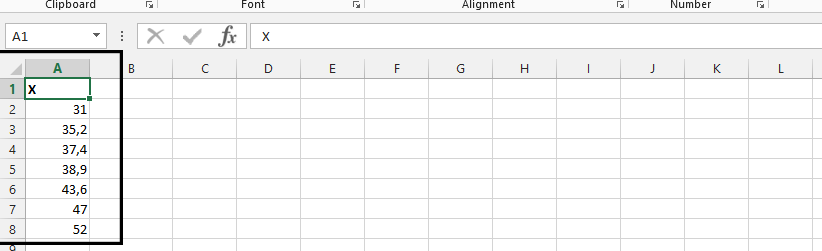Click on home tab.Click on a value in the data, and Sort & filter to choose Sort from smallest to largest.Start another column, with one in between, and label it i.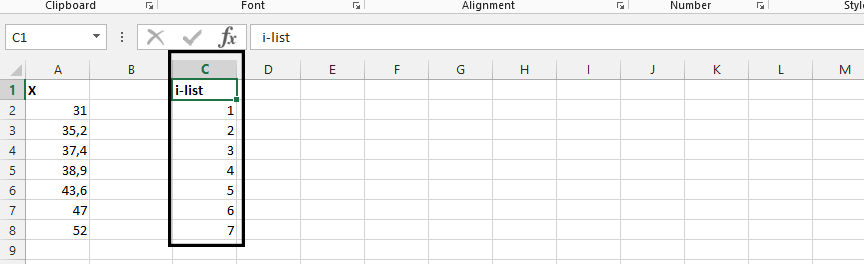Start another column, and label it formula.Click on D2 (1), and type

=(C2-0,375)/(6+0,25)(2), and enterDouble click on the small square in the right bottom corner of the cell.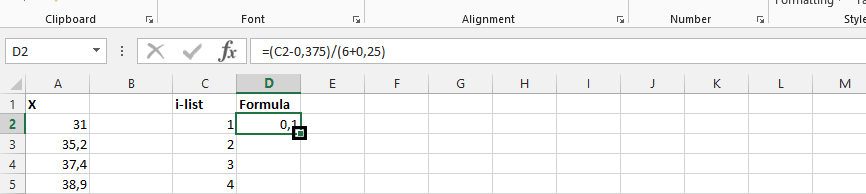Label Column B Expected.Click on B2 (1), and type

=NORM.S.INV(D2) (2)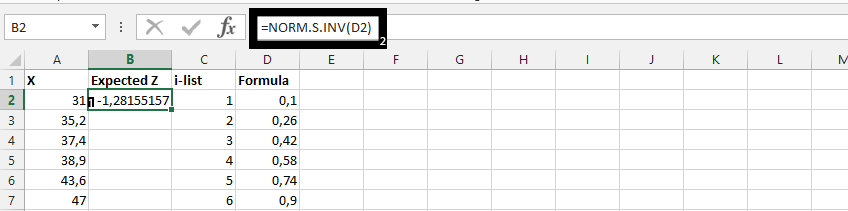Double click on the small square showing in the result from previous step.Highlight A2-B8 (1), click on insert (2), scatter chart (3), and choose desired chart (4) to plot normal distribution chart.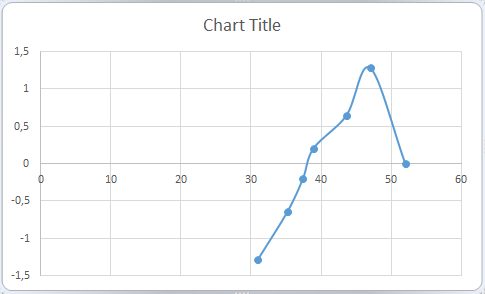In conclusion, in the picture above, you see #NUM! This means the formula in D8 is above 1, and therefore not possible to be part of the probability.

## Template

```Further reading: